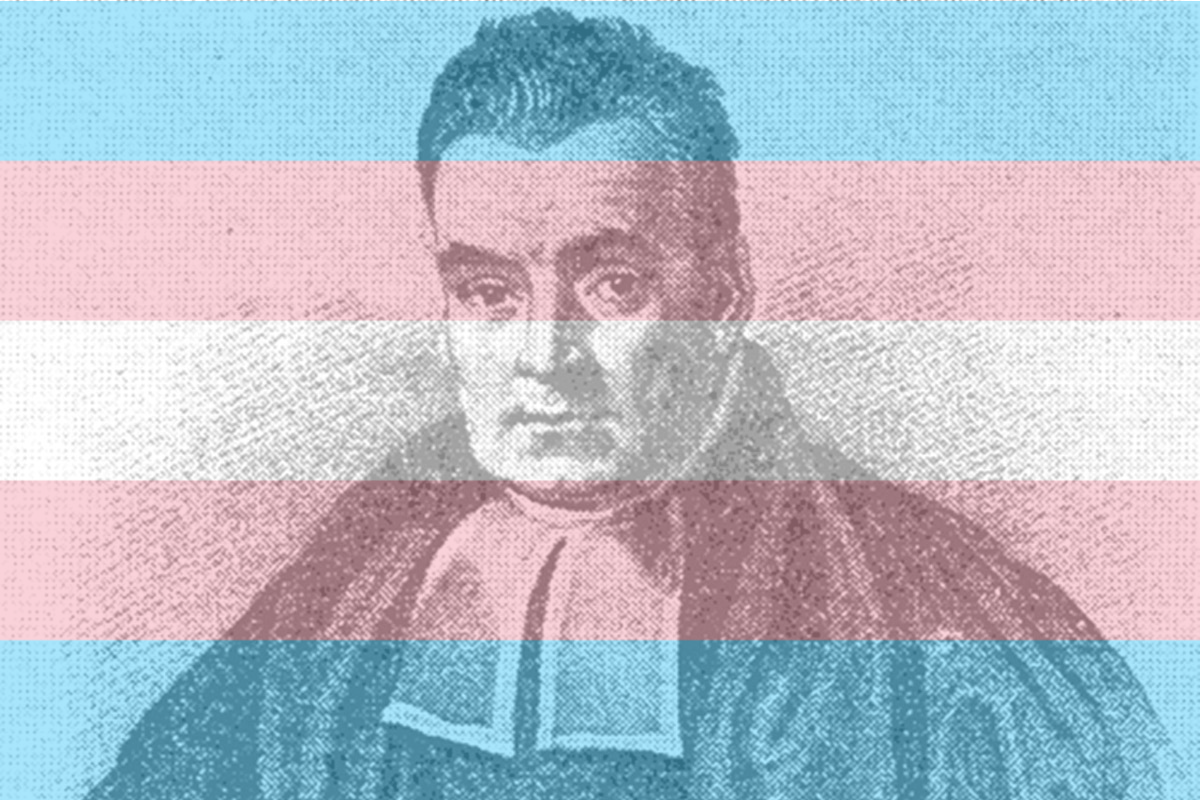# Shae's Ramblings

Stuff I find slightly meaningful and close to achievable

## Thomas Bayes can tell you if you're TransgenderAs some of you may know, I'm autistic. Nothing major, just a few things I do differently on a daily basis, and a few abilities I have others don't and vice-versa.

My therapist sent me this Nature Comms article about gender identity and autism two months ago, and I was mildly annoyed that every quantity was reported as an Odds-Ratio and not just a probability $p\in (0,1)$.

Some results are harder to parse this way, such as:

Transgender and gender-diverse individuals had higher rates of autism diagnosis compared to cisgender males (OR = 4.21, 95%CI = 3.85–4.60, p value $< 2 × 10^{−16}$, cisgender females (OR = 6.80, 95%CI = 6.22–7.42, p value$< 2 × 10^{−16}$, and cisgender individuals altogether (i.e., cisgender males and cisgender females combined) (OR = 5.53, 95%CI = 5.06–6.04, p value$< 2 × 10^{−16}$

So how do we translate such a thing as $\text{OR} = 4.21$ ? Let's find out.

## Crunching the numbers

To interpret this statement

Transgender and gender-diverse individuals had higher rates of autism diagnosis compared to cisgender males (OR = 4.21, 95%CI = 3.85–4.60, p value $< 2 × 10^{−16}$, cisgender females (OR = 6.80, 95%CI = 6.22–7.42, p value$< 2 × 10^{−16}$, and cisgender individuals altogether (i.e., cisgender males and cisgender females combined) (OR = 5.53, 95%CI = 5.06–6.04, p value$< 2 × 10^{−16}$

we should look at the methodology described in the second figure's legend: we should interpret

$$\text{OR} = p/q = \dfrac{\mathbb{P}(\text{Autism}\mid \text{GD}) }{\mathbb{P}(\text{Autism}\mid \lnot\text{GD})}$$

where $\text{GD}$ corresponds to being gender-diverse or not.
Here $\lnot \text{GD}$ should be read as “not $\text{GD}$”, it is the logical negation: it means you're cisgender in short.

Further, in the introduction, they recall that

Approximately 1–2% of the general population is estimated to be autistic based on large-scale prevalence and surveillance studies

As well as

Currently, 0.4–1.3% of the general population is estimated to be transgender and gender-diverse, although the numbers vary considerably based on how the terms are defined

which boils down to $\mathbb{P}(\text{Autism}) \in (0.01, 0.02)$ and $\mathbb{P}(\text{GD}) \in (0.004, 0.013)$. We'll denote these $p_\text{A}$ and $p_\text{GD}$ for short.

We obviously have that $p_\text{GD}p + (1-p_\text{GD})q = p_\text{A}$.

That yields us (if we recall $p = \text{OR}\cdot q$ ) the values

$$q \triangleq \mathbb{P}(\text{Autism}\mid \lnot\text{GD}) = \dfrac{p_\text{A}}{1+p_\text{GD}(\text{OR}-1)} \approx \dfrac{0.015}{0.0085\cdot (4.2-1)} \approx 0.0146$$
If we just take the confidence intervals' midpoints.

This means, not accounting for uncertainty, that a cisgender person has a $1.45\%$ chance of being autistic, while a gender-diverse person has a much higher (4.2 times higher) chance at $6.13\%$ .

## Bayes' Rule and the egg question

Am I transgender or not ?

Is a question I ask myself often, and others too. But right now, my question is a tiny bit more specific. It is rather:

Am I transgender, given that I already know I'm autistic ?

Answering this question boils down to estimation of $p_\text{egg} \triangleq\mathbb{P}(\text{GD} \mid \text{Autism})$.

This is where our dear friend reverend Thomas Bayes can help us !

According to his findings, this is simply

$$p_\text{egg} = \frac{ \mathbb{P}(\text{Autism}\mid \text{GD}) \mathbb{P}(\text{GD})}{\mathbb{P}(\text{Autism})} = p\cdot p_\text{GD} / p_\text{A} \approx 0.03479$$

Turns out, as an autistic person, I have a $3.48\%$ chance of being transgender !

This is much higher than the upper bound of $1.3\%$ for the average joe.

## Confidence intervals

This is just to have a point prediction, but how do we compute the confidence intervals ?

Turns out it's not that easy, and this is the main focus of an entire other blogpost.

After one night of coding and pretty intricate hacks, I am proud to report that I can estimate any of the paper's probabilities.

As an example, here are a few entries:

Control group Dataset $\mu$ $\sigma$ Probability Type $95\%$ Confidence Interval
cisgender-individuals-altogether MU $-2.451876$ $0.522116$ $0.098709$ $\mathbb{P}(\text{Autism}\mid\text{GD})$ $0.030954, 0.239661$
cisgender-individuals-altogether MU $-4.398846$ $0.485557$ $0.0138293$ $\mathbb{P}(\text{Autism}\mid\lnot\text{GD})$ $0.004745, 0.031836$

## Computing all the paper's probabilities

I am a man (woman?) of my word, so here is the entire paper's dataset converted to 0-100 probabilities.

But let's visualize some neat things, shall we ?

Here are the exact results from the paper, reframed in terms of probabilities:But wait ! I can do more : here is the entire distribution of possible probability values for $\mathbb{P}(\text{Autism}\mid\text{GD})$As you can see, that $6.13\%$ estimate is pretty conservative ! It can go as high as $10\% +$ with high likelihood.

## Back to the “Egg question”

What about the probabilities of being trans assuming I'm autistic ?

Here they are:We can see I have a much higher chance of being transgender, but nothing north of the range $5-10\%$.

Voilà, that concludes our little data escapade :)